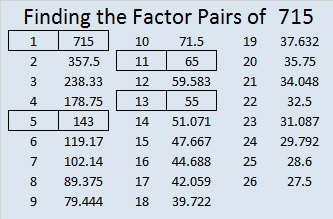# 715 and Level 5

• 715 is a composite number.
• Prime factorization: 715 = 5 x 11 x 13
• The exponents in the prime factorization are 1, 1, and 1. Adding one to each and multiplying we get (1 + 1)(1 + 1)(1 + 1) = 2 x 2 x 2 = 8. Therefore 715 has exactly 8 factors.
• Factors of 715: 1, 5, 11, 13, 55, 65, 143, 715
• Factor pairs: 715 = 1 x 715, 5 x 143, 11 x 65, or 13 x 55
• 715 has no square factors that allow its square root to be simplified. √715 ≈ 26.73948.Can you solve today’s puzzle?Print the puzzles or type the solution on this excel file: 12 Factors 2015-12-14

————————————————————————————

Here are several more facts about the number 715:

715 is the 22nd pentagonal number because 22(3⋅22 – 1)/2 = 715.

715 can be written as the sum of consecutive numbers several ways:

• 357 +358 = 715; that’s 2 consecutive numbers.
• 141 + 142 + 143 + 144 + 145 = 715; that’s 5 consecutive numbers.
• 60 + 61 + 62 + 63 + 64 + 65 + 66 + 67 + 68 + 69 + 70 = 715; that’s 11 consecutive numbers.
• 49 + 50 + 51 + 52 + 53 + 54 + 55 + 56 + 57 + 58 + 59 + 60 + 61 = 715; that’s 13 consecutive numbers.

Since 5 and 13 are two of its factors, 715 is the hypotenuse of four Pythagorean triples.

• 176-693-715
• 275-660-715
• 363-616-715
• 429-572-715

Each of those triples has a greatest common factor. Can you find them all?

715 is also palindrome 1D1 in BASE 21 where D = 13 base 10; note that 1(21²) + 13(21) + 1(1) = 715.

————————————————————————————